Which of the following velocity-time graphs represent uniform motion

 (1)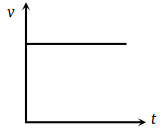(2)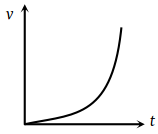(3)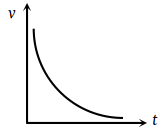(4)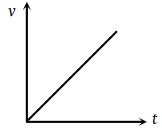Concept Questions :-

Graphs
High Yielding Test Series + Question Bank - NEET 2020

Difficulty Level:

Acceleration-time graph of a body is shown. The corresponding velocity-time graph of the same body is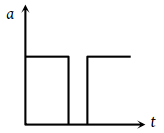(1)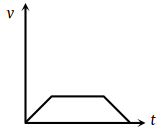(2)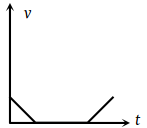(3)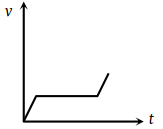(4)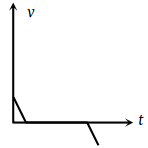Concept Questions :-

Graphs
High Yielding Test Series + Question Bank - NEET 2020

Difficulty Level:

The given graph shows the variation of velocity with displacement. Which one of the graphs given below correctly represents the variation of acceleration with displacement ?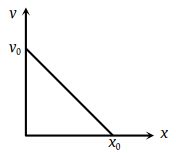1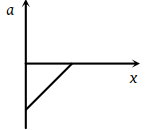2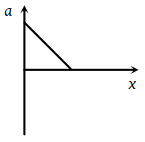3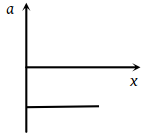4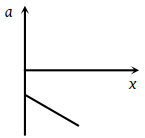Concept Questions :-

Graphs
High Yielding Test Series + Question Bank - NEET 2020

Difficulty Level:

The acceleration-time graph of a body is shown below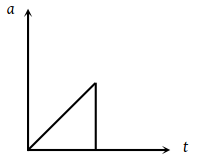The most probable velocity-time graph of the body is

 (1)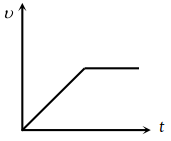(2)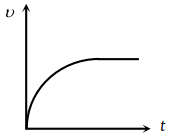(3)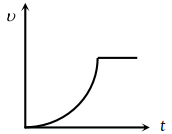(4)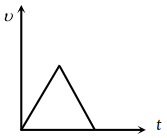Concept Questions :-

Graphs
High Yielding Test Series + Question Bank - NEET 2020

Difficulty Level:

From the following displacement-time graph , find out the velocity of a moving body.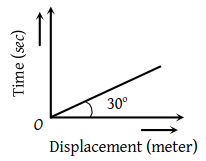1. $\frac{1}{\sqrt{3}}$m/s

2. 3 m/s

3. $\sqrt{3}$m/s

4. $\frac{1}{3}$m/s

Concept Questions :-

Graphs
High Yielding Test Series + Question Bank - NEET 2020

Difficulty Level:

The vt plot of a moving object is shown in the figure. The average velocity of the object during the first 10 seconds is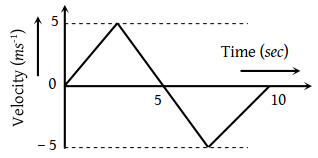(1) 0

(2) 2.5 ms–1

(3) 5 ms–1

(4) 2 ms–1

Concept Questions :-

Graphs
High Yielding Test Series + Question Bank - NEET 2020

Difficulty Level:

Read the assertion and reason carefully to mark the correct option out of the options given below:

(1) If both assertion and reason are true and the reason is the correct explanation of the assertion.

(2) If both assertion and reason are true but reason is not the correct explanation of the assertion.

(3) If assertion is true but reason is false.

(4) If the assertion and reason both are false.

(5) If assertion is false but reason is true.

Assertion : A body can have acceleration even if its velocity is zero at a given instant of time.

Reason : A body is momentarily at rest when it reverses its direction of motion.

Concept Questions :-

Instantaneous speed and instantaneous velocity
High Yielding Test Series + Question Bank - NEET 2020

Difficulty Level:

Read the assertion and reason carefully to mark the correct option out of the options given below:

(1) If both assertion and reason are true and the reason is the correct explanation of the assertion.

(2) If both assertion and reason are true but reason is not the correct explanation of the assertion.

(3) If assertion is true but reason is false.

(4) If the assertion and reason both are false.

(5) If assertion is false but reason is true.

Assertion : Two balls of different masses are thrown vertically upward with same speed. They will pass through their point of projection in the downward direction with the same speed.

Reason : The maximum height and downward velocity attained at the point of projection are independent of the mass of the ball.

Concept Questions :-

Acceleration
High Yielding Test Series + Question Bank - NEET 2020

Difficulty Level:

Read the assertion and reason carefully to mark the correct option out of the options given below:

(1) If both assertion and reason are true and the reason is the correct explanation of the assertion.

(2) If both assertion and reason are true but reason is not the correct explanation of the assertion.

(3) If assertion is true but reason is false.

(4) If the assertion and reason both are false.

(5) If assertion is false but reason is true.

Assertion : If the displacement of the body is zero, the distance covered by it may not be zero.

Reason : Displacement is a vector quantity and distance is a scalar quantity.

Concept Questions :-

Distance and displacement
High Yielding Test Series + Question Bank - NEET 2020

Difficulty Level:

Read the assertion and reason carefully to mark the correct option out of the options given below:

(1) If both assertion and reason are true and the reason is the correct explanation of the assertion.

(2) If both assertion and reason are true but reason is not the correct explanation of the assertion.

(3) If assertion is true but reason is false.

(4) If the assertion and reason both are false.

(5) If assertion is false but reason is true.

Assertion : The average velocity of the object over an interval of time is either smaller than or equal to the average speed of the object over the same interval.

Reason : Velocity is a vector quantity and speed is a scalar quantity.

Concept Questions :-

Average speed and average velocity